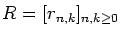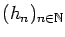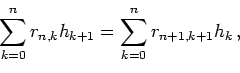Journal of Integer Sequences, Vol. 20 (2017), Article 17.8.2

## Shifting Property for Riordan, Sheffer and Connection Constants Matrices

### Emanuele Munarini Politecnico di Milano Dipartimento di Matematica Piazza Leonardo da Vinci 32 20133 Milano Italy

Abstract:

We study the shifting property of a matrixand a sequence, i.e., the identitywhen R is a Riordan matrix, a Sheffer matrix (exponential Riordan matrix), or a connection constants matrix (involving symmetric functions and continuants). Moreover, we consider the shifting identity for several sequences of combinatorial interest, such as the binomial coefficients, the polynomial coefficients, the Stirling numbers (and their q-analogues), the Lah numbers, the De Morgan numbers, the generalized Fibonacci numbers, the Bell numbers, the involutions numbers, the Chebyshev polynomials, the Stirling polynomials, the Hermite polynomials, the Gaussian coefficients, and the q-Fibonacci numbers.

Full version:  pdf,    dvi,    ps,    latex

Received September 28 2016; revised versions received October 3 2016; July 6 2017; August 24 2017; August 25 2017. Published in Journal of Integer Sequences, August 31 2017.